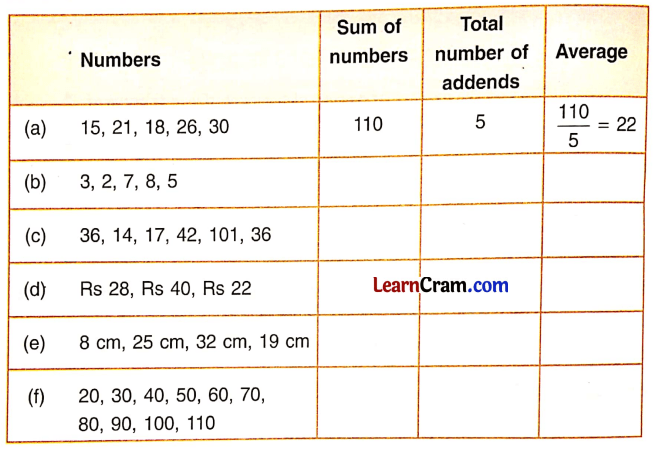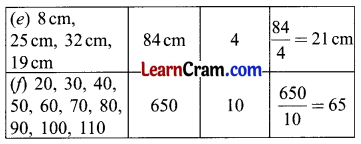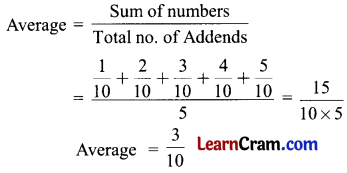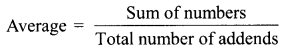# DAV Class 5 Maths Chapter 10 Worksheet 1 Solutions

The DAV Books Solutions Class 5 Maths and DAV Class 5 Maths Chapter 10 Worksheet 1 Solutions of Averages offer comprehensive answers to textbook questions.

## DAV Class 5 Maths Ch 10 Worksheet 1 Solutions

Question 1.
Complete the table by finding the average of these sets. The first one is done for you.Solution:Question 2.
Find the average of $$\frac{1}{10}, \frac{2}{10}, \frac{3}{10}, \frac{4}{10}, \frac{5}{10}$$.
Solution:Question 3.
Fill in the blanks:
(a) We divide 14 by ______ to find the average of 8 and 6.
Solution:
2

(b) The average of 11 and 9 is ______
Solution:
10

(c) The average of 10, 100 and 1,000 is ______
Solution:
370

(d) We divide ______ by ______ to find the average of 1, 2, 3, 14 and 10.
Solution:
30, 5

(e) The average of 16 kg, 18 kg, and 20kg is ______ kg.
Solution:
18

DAV Class 5 Maths Chapter 10 Worksheet 1 Notes

Division: It is the operation that carries out the distribution of things with each person or in 1 litre/1 kilogram.
The idea of average is not new to us. We all are familiar with the following types of statements:

• The average number of runs scored by Sachin Tendulkar in the series is 68.
• The average mark secured by Raghav is 83%. If we have a group of 4 students we have to divide 24 toffees to them equally. We count the toffees and divide the sum by the number of students then distribute it equally to them, e.g. 24 ÷ 4 = 6. Each child gets 6 toffees.

To find the average of a group of numbers, divide the sum of numbers by the total number of addends.Example:
Shyam is a vegetable seller. His daily earnings for seven days are ₹ 20, ₹ 26, ₹ 32, ₹ 25, ₹ 27, ₹ 24, ₹ 28. What must be his average daily earnings?
Solution:
Total earning of Shyam for seven days = ₹ 20 + ₹ 26 + ₹ 32 + ₹ 25 + ₹ 27 + ₹ 24 + ₹ 28 = ₹ 182
Number of days = 7
Average daily earning = ₹ 182 ÷ 7 = ₹ 26
Shyam’s average daily earning is ₹ 26.